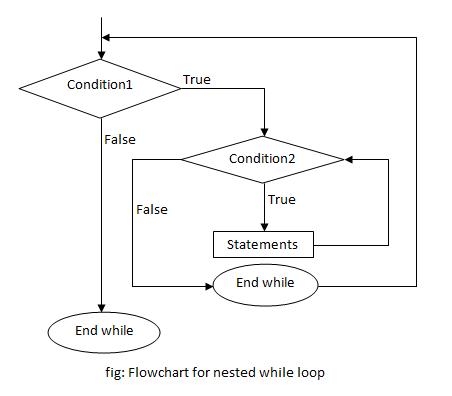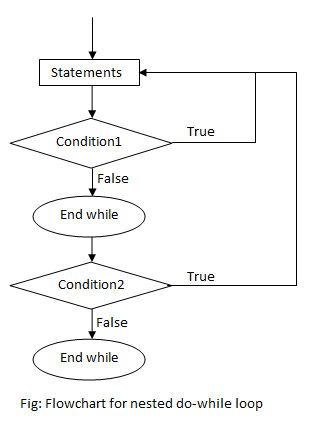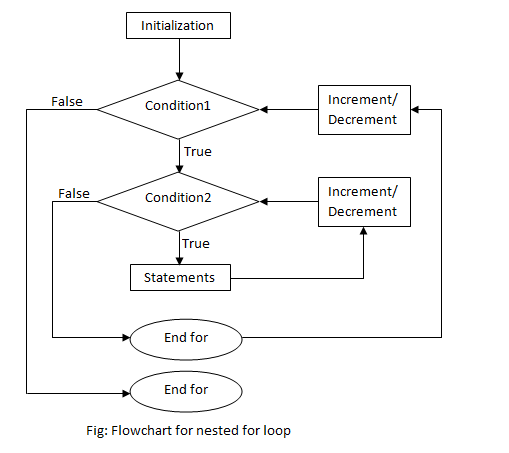# Nested loop in C

A loop inside another loop is called a nested loop. The depth of nested loop depends on the complexity of a problem. We can have any number of nested loops as required. Consider a nested loop where the outer loop runs n times and consists of another loop inside it. The inner loop runs m times. Then, the total number of times the inner loop runs during the program execution is n*m.

## Types of nested loops

Note: There can be mixed type of nested loop i.e. a for loop inside a while loop, or a while loop inside a do-while loop.

## Nested while loop

A while loop inside another while loop is called nested while loop.

### Syntax of Nested while loop

```while (condition1)
{
statement(s);
while (condition2)
{
statement(s);
... ... ...
}
... ... ...
}```

### Flowchart of Nested while loop### Example of Nested while loop

Example 1: C program to print the number pattern.

```1
1 2
1 2 3
1 2 3 4
1 2 3 4 5```
```#include <stdio.h>
int main()
{
int i=1,j;
while (i <= 5)
{
j=1;
while (j <= i )
{
printf("%d ",j);
j++;
}
printf("n");
i++;
}
return 0;
}```

In this program, nested while loop is used to print the pattern. The outermost loop runs 5 times and for every loop, the innermost loop runs i times which is 1 at first, meaning only “1” is printed, then on the next loop it’s 2 numbers printing “1 2” and so on till 5 iterations of the loop executes, printing “1 2 3 4 5”. This way, the given number pattern is printed.

## Nested do-while loop

A do-while loop inside another do-while loop is called nested do-while loop.

### Syntax of Nested do-while loop

```do
{
statement(s);
do
{
statement(s);
... ... ...
}while (condition2);
... ... ...
}while (condition1);```

### Flowchart of Nested do-while loop### Example of Nested do-while loop

Example 2: C program to print the given star pattern.

```*
**
***
****
*****```
```#include <stdio.h>
int main()
{
int i=1,j;
do
{
j=1;
do
{
printf("*");
j++;
}while(j <= i);
i++;
printf("n");
}while(i <= 5);
return 0;
}```

In this program, nested do-while loop is used to print the star pattern. The outermost loop runs 5 times and for every loop, the innermost loop runs i times which is 1 at first, meaning only one “*” is printed, then on the next loop it’s 2 printing two stars and so on till 5 iterations of the loop executes, printing five stars. This way, the given star pattern is printed.

## Nested for loop

A for loop inside another for loop is called nested for loop.

### Syntax of Nested for loop

```for (initialization; condition; increment/decrement)
{
statement(s);
for (initialization; condition; increment/decrement)
{
statement(s);
... ... ...
}
... ... ...
}```

### Flowchart of Nested for loop### Example of Nested for loop

Example 3: C program to print all the composite numbers from 2 to a certain number entered by user.

```#include<stdio.h>
#include<math.h>
int main()
{
int i,j,n;
printf("Enter a number:");
scanf("%d",&n);
for(i=2;i<=n;i++)
{
for(j=2;j<=(int)pow(i,0.5);j++)
{
if(i%j==0)
{
printf("%d is compositen",i);
break;
}
}
}
return 0;
}
```

Output

```Enter a number:15
4 is composite
6 is composite
8 is composite
9 is composite
10 is composite
12 is composite
14 is composite
15 is composite```

A number is said to be composite if it has at least one factor other than 1 and itself. This program prints all the composite numbers starting from 2 to a certain number n, entered by user. We need to use a nested loop to solve this problem. The outer for loop runs from 2 to n and the inner loop is used to determine whether a number is composite or not. We need to check for that factor starting from 2 to integer part of square root of that number.

Consider 15, its square root is nearly 3.873. Here, the integer part is 3. Now, if there is a factor of 15 from 2 to 3 then it is composite. Here, 3 is a factor of 15. Hence, 15 is a composite number.